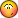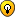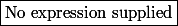# Intégrale - Breeden Litzenberger

Réponses à toutes vos questions après le Bac (Fac, Prépa, etc.)
NicoAix
Messages: 1
Enregistré le: 17 Mar 2016, 18:36

###Intégrale - Breeden Litzenberger

Bonjour à tous !

Je bloque complètement sur un exercice que j'ai à faire, à savoir que j'ai emprunté 2-3 livres à la BU, sans pour autant que ça ne m'avance à quelque choseC'est un blocage complet, mis à part la question 1 avec Black & Scholes. Si quelqu'un a des idées pour la suite, je suis preneurJe joins l'exercice :
https://www.fichier-xls.fr/2016/03/17/bl-work/

Breeden Litzenberger formula

Assume the risk neutral conditional distribution function of a stock S between time t and T (t < T) is noted Q(t, x, T, y). It gives the probability the stock value in T is y given that St = x in t.
Then the price of a call option is :Remark that :And :Then it means that if a continuum of market call option prices are available (for several strikes), we can compute the conditional distribution function from the second derivatives.

1. Build a call option pricer in BS framework. Choose a maturity T, r andChoose a set of strikes on [0, 3 × St] (maybe around N = 200 strikes), with St = x = 100 for example. Price the N calls.

2. Use the N prices to compute the second derivatives (with finite difference method) and compute the transition probabilities Q(t, x, T,K) for each element of the set of strikes (you can considere r = 0). Plot the histogram and comment this distribution. Compare to a lognormal distribution.

3. Compute the call prices from fomula (1) using a Riemann sum (rectangle or trapeze integration) and the probabilities computed previously.

4. Do the same work using different sets of strikes (a lot of strikes available or only a few). What can you say about the prices of calls obtained with the Riemann sum and their prices obtained from the BS closed formula (numerical unstability) ?

Retourner vers ✯✎ Supérieur

### Qui est en ligne

Utilisateurs parcourant ce forum : marawita1 et 23 invités

Tu pars déja ?

Fais toi aider gratuitement sur Maths-forum !

Créé un compte en 1 minute et pose ta question dans le forum ;-)

Identification

Pas encore inscrit ?

Ou identifiez-vous :